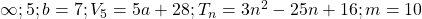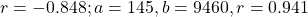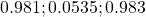# 2020 A-level H2 Mathematics (9758) Paper 2 Suggested SolutionsAll solutions here are suggested. Mr. Teng will hold no liability for any errors. Comments are entirely personal opinions.

Question 1:Question 2:Question 3:Question 4:Question 5:Question 6:Question 7:Question 8:Question 9:Question 10:•-

perhaps question 10 upper limit should be 100 since it’s percentage

•KS Teng

Thats true. Good point. Thanks.

•:)

Any thoughts on this year’s math papers?

•anon

when you scale x by factor 2, wouldnt x be (x/2) and hence area should be 1.5 times?

•KS Teng

the 2 way stretch results in.

•Alvin Pang

Why is there pi in 4(ii)? Isn’t it a square base though.

•KS Teng

•.

is qn 9iii 0.0535 instead of 0.535?

•KS Teng

indeed, copied wrongly off the GC. Thank you.

•hii

is qn 9iii 0.0535 instead of 0.535?

•KS Teng

indeed, copied wrongly off the GC. Thank you.

•-

9(iii) 0.0535?

•KS Teng

indeed, copied wrongly off the GC. Thank you.

•:)

should q9 be 0.0535 instead of 0.535?

•KS Teng

indeed, copied wrongly off the GC. Thank you.

•:>

for q3, isnt the limit for the area under the parametric eqn from t=1/6 to t=2, instead of from t=0.5? when y=0, t=1/6?

Not readable? Change text.